• PAGE 1 实验文件程序设计实验目的 1. 掌握文件的基本概念 2. 掌握文本文件的打开和关闭操作 3. 掌握文本文件的顺序读写方法 二实验要求 1.编写程序从键盘输入若干实数以特殊数值-1结束分别写到一个文本文件中...
• 标准 文案 桂林电子科技大学 数学与计算科学学院实验报告 院系 数学与计算科学 学号 姓名 成绩 课程 名称 数学应用软件实验 实验项目 名 称 实验三 MATLAB的程序设计 一 实验目的 1. 掌握建立与编辑函数文件和脚本...
• PAGE PAGE 1 实验三 MATLAB 程序设计 题目 1 编写M 函数实现求一个数是否为素数再编写一主程序脚本文件要求通过 键盘输入一个整数然后调用判断素数函数从而确定它是否素数 2 编写M 函数统计一数值中零的个数然后编写...
• 实验要求完成两个程序，文件名分别为：txt.c ---文本文件操作； binary.c ---二进制文件操作。程序具体功能要求如下： 1）从键盘读入数据到结构体数组：调用Input()函数输入学生信息，结构体定义题目已经给出，...
• 班级号 _学号_姓名_题号 .java(多文件应压缩为 rar) 实验 1: 熟悉上机环境编写并运行简单的 java 程序 3 学时 实验目的 (1) 熟悉 Java 程序开发环境 J2SDK+JCreator 的安装及使用 (2) 熟悉 Java Application 和 ...
• 计算等式 三位数个十百位交换 计算1-100内的素数计算a^b式乘方 猜数游戏 汉诺塔 学生成绩管理表 文件操作
• Matlab 程序设计基本方法 覃照乘 自092 电气工程学院 一 实验目的 1熟悉 MATLAB 程序编辑与设计环境 2掌握各种编程语句语法规则及程序设计方法 3函数文件的编写和设计 4 了解和熟悉跨空间变量传递和赋值 二实验基本...
• 本次课程设计使用C语言制作程序来实现图书的登记，删除，查询，浏览以及读者的借阅，还书，会员登录，修改密码等功能。程序中涉及到数据链表和文件指针的操作，包括结构体数据定义，使用及文件的读写，定位，修改等...
C课程设计——图书管理系统
1、题目意义
图书馆，作为文献的聚集地和展示平台，常常扮演着引领文化前进的角色，是每个大学不可或缺的基础设施，而图书管理系统则是一个图书馆能够正常运转的关键。本次课程设计使用C语言制作程序来实现图书的登记，删除，查询，浏览以及读者的借阅，还书，会员登录，修改密码等功能。程序中涉及到数据链表和文件指针的操作，包括结构体数据定义，使用及文件的读写，定位，修改等。
2、设计思想
①新建账号密码，然后通过二者的匹配登录系统，这样可以保证是本校同学才能使用，另外还可以修改密码。
②添加图书的基本信息，如 书号，书名，作者，出版社，类别，进库量；
③删除图书，通过图书的书名进行删除；
④图书查询，通过图书的书号，书名，作者或者类别进行检索；
⑤浏览图书的库存，将所有数目展示出来；
⑥借书登记，先判断是否有这本数，然后进行借书人学号，姓名，归还截止期限，借书书名进行登记
⑦还书情况查看。
⑧借阅情况查看。
⑨会员登录，通过数据文件中的已注册的账号密码来登录到图书管理系统。
⑩修改密码
3.总设计框架图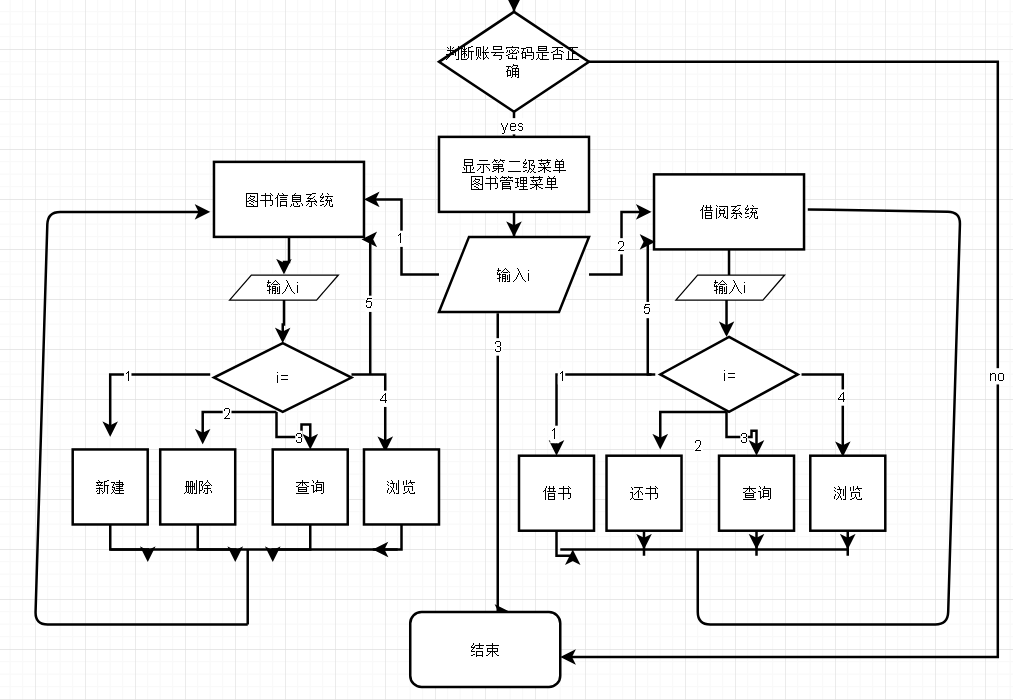4.代码展示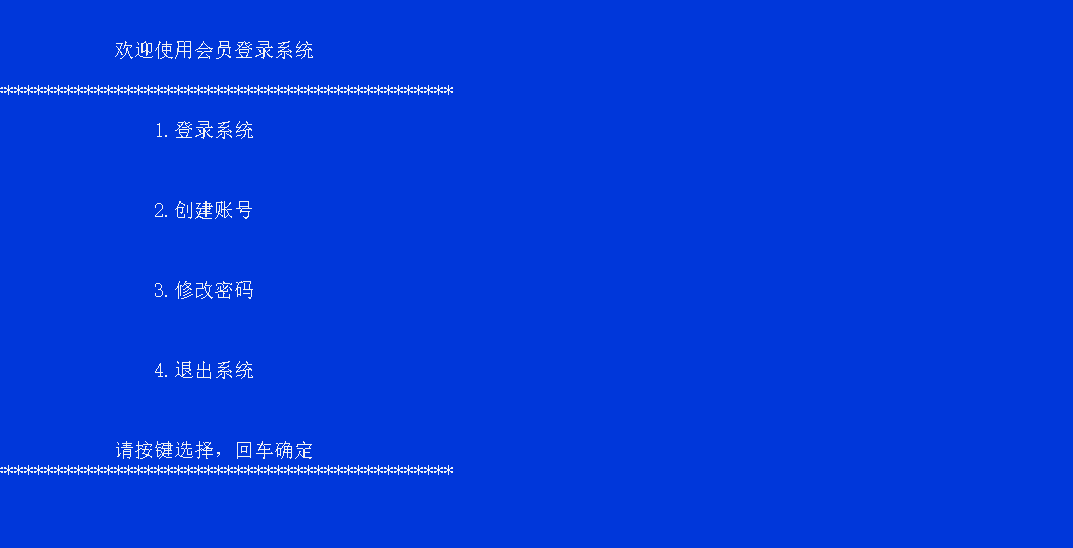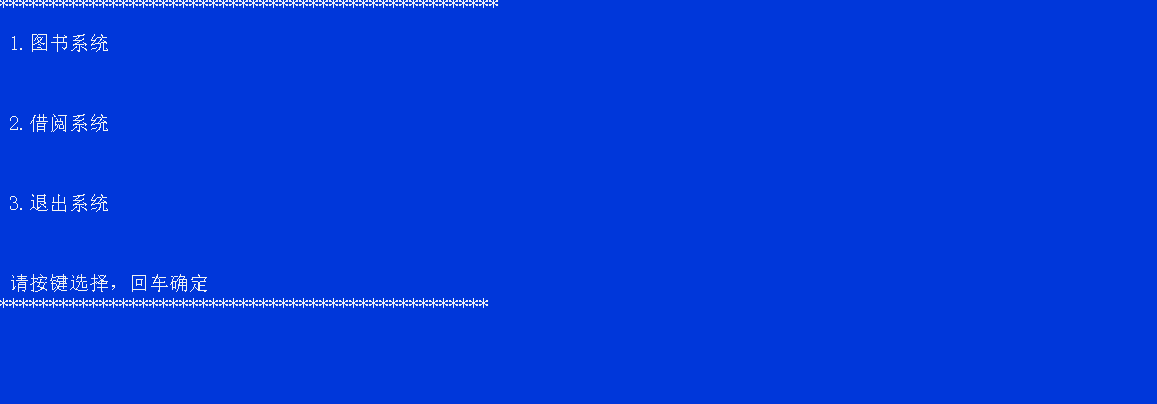#include <stdio.h>
#include <stdlib.h>//其中包含system函数
#include <conio.h>//定义了通过控制台进行 数据输入 和数据输出的函数，如getch函数。
#include <string.h>//定义字符数组
#include <math.h>
#define LEN sizeof(struct book)//有关图书信息的结构体
#define LEN1 sizeof(struct reader)//有关读者信息的结构体
#define LEN2 sizeof(struct land)//有关登录系统的结构体

struct book//图书信息
{
char num,nam,aut,pub,cat;
int  many;
float price;
struct book*next;
};

struct reader//读者借阅信息
{
int jnum;
char jnam,time,tsnam;
struct reader *next;
};
struct land//登录信息
{
int zhanghao;
char password;
struct land*next;
};

int tjzs();//统计library文本个数函数
void xg(int,char[] );
void mainmenu();//显示主菜单
void tsmenu();//显示图书信息菜单
void jmenu();//显示借阅信息菜单
void lmenu();//显示登录菜单

void main1();// 主菜单功能
void tsmain();//图书菜单功能
void cxts();//查询图书
void zjts();//增加图书
void scts();//删除图书
void llts();//浏览图书

void jmain();//读者借阅信息
void js();//借书
void hs();//还书
void cxjs();//查询借书
void lljs();// 浏览借书情况

void land();//登录功能系统
int xinjian(int,char[] );//创建账号密码
void xgmm();//修改密码
void lmain();//登录界面函数

int tjzs()//统计图书文本个数
{
FILE *fp;
int txcl=0,n;
float tprice=0;
char tname={'\0'},tauthor={'\0'},tchuban={'\0'},tkind={'\0'},tshuhao={'\0'};
fp=fopen("library.txt","r");//打开文件
for (n=0;!feof(fp);n++)//逐个读文件
fscanf(fp,"%s%s%s%s%s%d%f",tshuhao,tname,tauthor,tchuban,tkind,&txcl,&tprice);
n--;
fclose(fp);//关闭文件
return (n);//返回个数
}

int tjdzzs()//统计文读者本个数函数

{
FILE *fp;
int zhenghao=0,n;
char mingzi={'\0'},riqi={'\0'},zname={'\0'};
fp=fopen("reader.txt","r");//打开文件
for (n=0;!feof(fp);n++)//逐个读文件
fscanf(fp,"%d%s%s%s ",&zhenghao,&mingzi,&riqi,&zname);
n--;
fclose(fp);//关闭文件
return (n);//返回个数
}

int tjzs3()//统计账号密码文本个数
{
FILE *fp;
int zhao=0,n;
char mm={'\0'};
fp=fopen("land.txt","r");//打开文件
for (n=0;!feof(fp);n++)//逐个读文件
fscanf(fp,"%d %s",&zhao,mm);
n--;
fclose(fp);//关闭文件
return (n);//返回个数
}

void mainmenu()//主菜单
{
system ("cls");

printf("**************************************************");

printf("\n\n 1.图书系统\n\n");

printf("\n\n 2.借阅系统\n\n");

printf("\n\n 3.退出系统\n\n");

printf("\n\n 请按键选择，回车确定\n");

printf("*************************************************\n");

return ;
}

void tsmenu() //图书馆信息菜单
{
system ("cls");

printf("****************************************************");

printf("\n 1.增加图书\n\n");

printf("\n 2.删除图书\n\n");

printf("\n 3.查询图书\n\n");

printf("\n 4.库存浏览\n\n");

printf("\n 5.返回上一层\n\n");

printf("\n 请按键选择，回车确定\n");

printf("***************************************************\n");

return ;

}

void main1()
{
void tsmian();
void jmain();
char choose;
mainmenu();
scanf(" %c",&choose);
switch(choose)//功能函数
{
case'1':
tsmain();
break;
case'2':
jmain();
break;
case'3':
system("cls");
getch();
exit(0);
system ("cls");
break;
}
}

void tsmain()
{
void zjts();
void scts();
void ctts();
void llts();
char choose;
tsmenu();
scanf(" %c",&choose);
for(;;)
{
switch(choose)
{
case'1':
zjts();
break;
case'2':
scts();
break;
case'3':
cxts();
break;
case'4':
llts();
break;
case'5':
main1();
break;
}
}
}

void zjts()//增加图书
{
FILE*fp;
char i;
int many=0;
float price=0;
char  nam={'\0'},aut={'\0'},cat={'\0'},pub={'\0'},num={'\0'};
system ("cls");

if ((fp=fopen("library.txt","r"))==NULL)//if语句：打开图书馆文件，不存在此文件则新建
{
fp=fopen("library.txt","w");
fclose(fp);
}
fp=fopen("library.txt","a");

printf("\n请按以下格式输入图书信息:\n书号 书名 作者 出版社 类别 进库量 单价");

for(;i!=27;)//为了实现输入一次后按esc退出
{
printf("请输入:\n");
scanf("%s%s%s%s%s%d%f",num,nam,aut,pub,cat,&many,&price);
fprintf(fp,"%-8s%-9s%-14s%-16s%-18s%-7d%-8.2f\n",num,nam,aut,pub,cat,many,price);
printf("继续输入请按回车，结束输入请按Esc\n");
i=getch();//暂停程序当i接收后继续下一条指令
for (;i!=13&&i!=27;)//保证只能是CR和ESC才能退出循环,输入其他字符无用，暂停程序，按'CR'继续。
i=getch();
}

fclose(fp);
printf("\n保存成功，按任意键返回上一层!");
getch();
tsmain();//返回上一层
}

void scts()//删除图书
{
struct book *head=NULL;
struct book *p,*p1,*p2;
int tmany=0,n=0,j,k;
float tprice=0;
char  tnam={'\0'},taut={'\0'},tcat={'\0'},tpub={'\0'},tnum={'\0'};
char jjnam={'\0'};
char i;
FILE *fp;
if ((fp=fopen("library.txt","r"))==NULL)//打开文件
{
system ("cls");
printf("\n记录文件不存在!按任意键返回");
getch();
tsmain();
}

else//实现删除的功能
{
system ("cls");
printf("\n请输入你要删除的书名:");//输入删除图书书名
scanf("%s",jjnam);
printf("\n确认删除请回车，取消请按Esc\n");
i=getch();
for(;i!=13&&i!=27;)
i=getch();
if (i==27)
tsmain();
fp=fopen("library.txt","r");
j=tjzs();

for (k=0;k<j;k++)
{
fscanf(fp,"%s%s%s%s%s%d%f",tnum,tnam,taut,tpub,tcat,&tmany,&tprice);
if (strcmp(jjnam,tnam))//比较名字，将不同名字的信息复制到链表
{
n++;//相同返回值为0不执行if语句继续循环，不同则执行直到将所有不同的书名建立成链表
if (n==1)//建立链表
{
p1=p2=(struct book*)malloc(LEN);
head=p1;
}
else
{
p2->next=p1;
p2=p1;
p1=(struct book*)malloc(LEN);//新建链表
}

strcpy(p1->num,tnum);//复制书号
strcpy(p1->nam,tnam);//复制书名
strcpy(p1->aut,taut);//复制作者名字
strcpy(p1->pub,tpub);//复制出版社
strcpy(p1->cat,tcat);//复制类别
p1->many=tmany;//复制个数
p1->price=tprice;//复制单价
}
}
if (n==0)//如果图书只有一项且这一项刚好和要删除的相同
{
head=NULL;
}
else//建立链表的最后剩余一个储存空间，所以封底
{
p2->next=p1;
p1->next=NULL;
fclose(fp);
}
}

fp=fopen("library.txt","w");//清空文件，只写打开，然后关闭
fclose(fp);
fp=fopen("library.txt","a");//追加文件
p=head;

for (;p!=NULL;)//把链表内容覆盖到文件
{
fprintf(fp,"%-8s%-9s%-14s%-16s%-18s%-7d%-8.2f\n",p->num,p->nam,p->aut,p->pub,p->cat,p->many,p->price);
p=p->next;
}
fclose(fp);
system ("cls");
printf("\n删除成功 \n按任意键返回上一层\n");
getch();//返回上一层
tsmain();
}

void cxts()//查询图书
{
FILE *fp;
int k=0,many=0,m=0,n=0;
float price=0;
char  nam={'\0'},aut={'\0'},cat={'\0'},pub={'\0'},num={'\0'};
char i;
char chazhao={'\0'};
if ((fp=fopen("library.txt","r"))==NULL)//打开文件
{
system ("cls");
printf("\n记录文件不存在!按任意键返回");
getch();
tsmain();
}
system("cls");
printf("请输入书号,书名,作者或类别查询：\n");
scanf("%s",chazhao);
system ("cls");
m=tjzs();

for (n=0;n<m;n++)
{
fscanf(fp,"%s%s%s%s%s%d%f",num,nam,aut,pub,cat,&many,&price);
if(!strcmp(chazhao,num)||!strcmp(chazhao,nam)||!strcmp(chazhao,aut)||!strcmp(chazhao,cat))
{
if(k==0)
{
printf("查询结果:\n\n");
printf("书号\t书名\t作者\t\t出版社\t\t类别\t\t现存量\t单价\n");
}
printf("%-8s%-9s%-14s%-16s%-18s%-7d%-8.2f\n",num,nam,aut,pub,cat,many,price);
k++;
}
}
if (k==0)//文件夹为空则输出无记录并返回上一层
{ system ("cls");
printf("\n无符合记录!\n");
getch();
tsmain();
}
fclose(fp);//查询结束
getch();
tsmain();
}

void llts()//浏览图书
{
FILE *fp;
int n=0;
int k=0,m=0,many=0;
float price=0;
char  nam={'\0'},aut={'\0'},cat={'\0'},pub={'\0'},num={'\0'};
char i;
if ((fp=fopen("library.txt","r"))==NULL)//打开文件
{
system ("cls");
printf("\n记录文件不存在!按任意键返回");
getch();//返回
tsmain();
}
n= tjzs();
if (n==0)
{
system ("cls");
printf("\n无任何记录!");
}

fp=fopen("library.txt","r");//打开只读文件
system ("cls");
printf("书号\t书名\t作者\t\t出版社\t\t类别\t\t库存量\t单价\n");

for (m=0;m<n;m++)//输出数据
{
fscanf(fp,"%s%s%s%s%s%d%f",num,nam,aut,pub,cat,&many,&price);
printf("%-8s%-9s%-14s%-16s%-18s%-7d%-8.2f\n",num,nam,aut,pub,cat,many,price);
}
fclose(fp);
printf("\n按任意键返回\n");
getch();
tsmain();
}

//~~~~~~~~~~~~~~~~~~~~~~~~~~~~~~~~~~~~~~~~~~~~~~~~~~~~~~~~~~~~~~~~~~~~~~~~~~~~~~~~~~~~~~~~~~~~~~~~~~~~~~~!~~~~~~~~~~~~~~~~~~~~~

void jmenu()//显示借书菜单
{
system("cls");
printf("*****************************************************");
printf("\n\n 1.借书登记\n\n");
printf("\n\n 2.还书登记\n\n");
printf("\n\n 3.借阅情况查看\n\n");
printf("\n\n 4.查询借书\n\n");
printf("\n\n 5.返回上一层\n\n");
printf("\n\n 请按键选择，回车确定\n");
printf("****************************************************");
return ;
}

void jmain()//借阅系统函数
{
void js();
void hs();
void lljs();
void cxjs();
char choose;
jmenu();
scanf(" %c",&choose);
for(;;)
{
switch(choose)
{
case'1':
js();
break;
case'2':
hs();
break;
case'3':
lljs();
break;
case'4':
cxjs();
break;
case'5':
main1();
break;
}
}

}

void js()//借书函数
{
FILE *fp,*fp3;
struct book *head=NULL;
struct book *p,*p1,*p2;
int i,loop,k,n=0,flag=0,s=0;
int jnum=0;
int many=0;
float tprice=0;
char tname={'\0'},tauthor={'\0'},tchuban={'\0'},tkind={'\0'},tshuhao={'\0'},
ttname={'\0'},mingzi={'\0'},riqi={'\0'},zname={'\0'};
char hit=0;

system("cls");
if((fp=fopen("library.txt","r"))==NULL)
{
system("cls");
printf("\n 图书馆无库存!按任意键退出!");
getch();
exit(0);
}
else
{
printf("\n请输入借阅书名:\n请输入:");
scanf("%s",zname);
k= tjzs();//统计图书馆文件个数

for (i=0;i<k;i++)//先将图书信息建立链表，更改库存
{
fscanf(fp,"%s%s%s%s%s%d%f",tshuhao,tname,tauthor,tchuban,tkind,&many,&tprice);
n++;
if(n==1)
{
p1=p2=(struct book*)malloc(LEN);
head=p1;
}
else
{
p2->next=p1;
p2=p1;
p1=(struct book*)malloc(LEN);

}
strcpy(p1->num,tshuhao);//复制书号
strcpy(p1->nam,tname);//复制书名
strcpy(p1->aut,tauthor);//复制作者
strcpy(p1->pub,tchuban);//复制出版社
strcpy(p1->cat,tkind);//复制类别
p1->many=many;//复制现存量
p1->price=tprice;//复制单价
}
if(n==0)
head=NULL;
else
{
p2->next=p1;
p1->next=NULL;
fclose(fp);
}

}
p=head;

for (;p!=NULL;)
{
if(!(strcmp(p->nam,zname)))//判断要借书的是否存在，标记等于1，存在库存减一
{

flag=1;
loop=p->many;
(p->many)--;
}
p=p->next;
}
if(flag&&(loop>0))//存在被借的图书且现存量大于0，把库存量变化后的链表存入文件
{

fp=fopen("library.txt","w");
fclose(fp);
fp=fopen("library.txt","a");
p=head;

for(;p !=NULL;)
{
fprintf(fp,"%-8s%-9s%-14s%-16s%-18s%-7d%-8.2f\n",p->num,p->nam,p->aut,p->pub,p->cat,p->many,p->price);
p=p->next;
}
free(p);
fclose(fp);

if ((fp3=fopen("reader.txt","r"))==NULL)//建读者文件夹存入借书信息
{
fp3=fopen("reader.txt","w");//打开只读文件
fclose(fp3);
}
fp3=fopen("reader.txt","a");

printf("\n请按以下格式输入读者信息:\n 证号 姓名 借书日期 借书书名\n请输入:");//录入读者信息
scanf("%d %s %s %s",&jnum,mingzi,riqi,zname);
fprintf(fp3,"\n%-8d%-23s%-18s%-10s\n",jnum,mingzi,riqi,zname);
fclose(fp3);
printf("借书成功!请两个月内归还！！！按任意键返回\n");
getch();//返回
jmain();//调用借阅系统
}
else
{
if(flag!=0)
printf("此书已被借完!按任意键返回!");//否则输出此书已被借完
else
printf("查找无此书!按任意键返回");
}
getch();//返回
jmain();//调用借阅系统

}

void hs ()//还书函数
{
FILE *fp,*fp3;
struct reader *head=NULL;
struct reader *p,*p1,*p2;
struct book *lhead1=NULL;
struct book *zp1,*lp1,*lp2;
int txcl=0,i;
float tprice=0;
char tname={'\0'},tauthor={'\0'},tkind={'\0'},
tchuban={'\0'},ttname={'\0'},tshuhao={'\0'};
int ttzhenghao=0,tzhenghao=0,n=0,k=0,t=0,flag=0;
char tmingzi={'\0'},triqi={'\0'},tzname={'\0'},ttzname={'\0'};
char hitkey=0;
system ("cls");
{
if ((fp=fopen("reader.txt","r"))==NULL)//不存在读者文件，则输出不能还书
{
system ("cls");
printf("\n 不存在借书者!按任意键退出!");
getch();
system("cls");
jmain();
}

else
{{
printf("\n请输入读者证号和书名:\n请输入:");
scanf("%d %s",&ttzhenghao,ttzname);//输入还书证号和书名
k=tjdzzs();//获取读者文件夹信息个数
for (i=0;i<k;i++)//读取读者文件夹信息
{
fscanf(fp,"%d%s%s%s\n ",&tzhenghao,tmingzi,triqi,tzname);
if((ttzhenghao==tzhenghao)&&!strcmp(ttzname,tzname))//如果证号书名存在，则标记为1
flag=1;
}
fclose(fp);
fp=fopen("reader.txt","r");//打开读者文件，删除借书信息
if(flag)
{
for (i=0;i<k;i++)//将读者文件复制到链表
{
fscanf(fp,"%d%s%s%s\n ",&tzhenghao,tmingzi,triqi,tzname);//读取文件信息
if(!((ttzhenghao==tzhenghao)&&!strcmp(ttzname,tzname)))
{
n++;
if (n==1)
{
p1=p2=(struct reader*)malloc(LEN1);//新建链表
head=p1;
}
else
{
p2->next=p1;
p2=p1;
p1=(struct reader*)malloc(LEN1);//新建链表
}
p1->jnum=tzhenghao;//复制证号
strcpy(p1->jnam,tmingzi);//复制读者名字
strcpy(p1->time,triqi);//复制日
strcpy(p1->tsnam,tzname);//复制书名
}}
if (n==0)
head=NULL;
else
{
p2->next=p1;
p1->next=NULL;
fclose(fp);
}
fp=fopen("reader.txt","w");//清空读者文件
fclose(fp);
fp=fopen("reader.txt","a");//追加信息
p=head;
for (;p!=NULL;)//把链表内容覆盖读者文件
{
fprintf(fp,"\n%-8d%-23s%-18s%-10s\n",p->jnum,p->jnam,p->time,p->tsnam);
p=p->next;
}
free(p);
fclose(fp);
}}}}

if(flag)//标记为1，即还书时更改库存
{{
{
printf("确认还书请按回车!");
for (;hitkey!=13&&hitkey!=27;)
hitkey=getch();
if (hitkey==13)
printf("成功!按任意键返回!");
n=0;flag=0;
fp3=fopen("library.txt","r");//打开图书馆文件
k=tjzs();//获取图书馆文件个数
for (i=0;i<k;i++)//将图书馆文件复制到链表
{
fscanf(fp3,"%s%s%s%s%s%d%f",tshuhao,tname,tauthor,tchuban,tkind,&txcl,&tprice);//读取信息
n++;
if (n==1)
{
lp1=lp2=(struct book*)malloc(LEN);//新建链表
lhead1=lp1;
}
else
{
lp2->next=lp1;
lp2=lp1;
lp1=(struct book*)malloc(LEN);//新建链表
}
strcpy(lp1->num,tshuhao);//复制书号
strcpy(lp1->nam,tname);//复制书名
strcpy(lp1->aut,tauthor);//复制作者
strcpy(lp1->pub,tchuban);//复制出版社
strcpy(lp1->cat,tkind);//复制类别
lp1->many=txcl; //复制现存量
lp1->price=tprice;//复制单价
}
if (n==0)
{
lhead1=NULL;
}
else
{
lp2->next=lp1;
lp1->next=NULL;
fclose(fp3);
}}}
zp1=lhead1;
for (;zp1!=NULL;)
{
if(!(strcmp(zp1->nam,ttzname)))//寻找书名相同
++(zp1->many);//现存量加1
zp1=zp1->next;
}
fp3=fopen("library.txt","w");//清空图书馆文件
fclose(fp);
fp3=fopen("library.txt","a");//追加信息
zp1=lhead1;
for (;zp1!=NULL;)//把链表内容覆盖图书馆文件
{
fprintf(fp3,"%-8s%-9s%-14s%-16s%-18s%-7d%-8.2f\n",
zp1->num,zp1->nam,zp1->aut,zp1->pub,zp1->cat,zp1->many,zp1->price);
zp1=zp1->next;
}
fclose(fp3);
getch();//返回
jmain();//调用借阅系统
}
else
printf("不存在此信息!按任意键返回!");
getch();//返回
jmain();//调用借阅系统
}

void lljs()//显示借书情况函数

{
FILE *fp;
int zhenghao=0,xcl=0,n=0,i=0,j=0;
char mingzi={'\0'},riqi={'\0'},zname={'\0'};
if ((fp=fopen("reader.txt","r"))==NULL)//打开读者文件夹
{
system ("cls");
printf("\n记录文件不存在!按任意键返回");
getch();
jmain();
}
n=tjdzzs();
if (n==0)
{ system ("cls");
printf("\n无任何记录!");
}
fp=fopen("reader.txt","r");
system ("cls");
printf("\n证号\t读者姓名\t\t借书日期\t书名\n");
for (;!feof(fp);)//输出文件信息
{
fscanf(fp,"%d%s%s%s\n ",&zhenghao,mingzi,riqi,zname);
printf("\n%-8d%-23s%-18s%-10s\n", zhenghao,mingzi,riqi,zname);
}
fclose(fp);
printf("\n按任意键返回\n");
getch();//返回
jmain();//调用借阅系统
}

void cxjs()//查询借书
{
FILE *fp;
int jsnum=0,k=0,many=0,m=0,n=0;

char  jsnam={'\0'},jstime={'\0'},tsnam={'\0'};
char i;
char chazhao={'\0'};
if ((fp=fopen("reader.txt","r"))==NULL)//打开文件
{
system ("cls");
printf("\n记录文件不存在!按任意键返回");
getch();
jmain();
}
system("cls");
printf("请输入证号或姓名查询：\n");
scanf("%s",chazhao);
system ("cls");
m=tjzs();
for (n=0;n<m;n++)
{
fscanf(fp,"%d%s%s%s",&jsnum,jsnam,jstime,tsnam);
if(!strcmp(chazhao,jsnam)||(('chazhao'-'0')==jsnum))
{
if(k==0)
{
printf("查询结果:\n\n");
printf("\n证号\t读者姓名\t\t借书日期\t书名\n");
}
printf("\n%-8d%-23s%-18s%-10s\n",jsnum,jsnam,jstime,tsnam);
k++;
}
}
if (k==0)//文件夹为空则输出无记录并返回上一层
{ system ("cls");
printf("\n无符合记录!\n");
getch();
jmain();
}
fclose(fp);//查询结束
getch();
jmain();
}

//~~~~~~~~~~~~~~~~~~~~~~~~~~~~~~~~~~~~~~~~~~~~~~~~~~~~~~~~~~~~~~~~~~~~~~~~~~~~~~~~~~~~~~~~~~~~~~~~~~~~~~~~~~~~~~~~~~~~~~~~~~~~~~~~~~~~~~

void lmenu()//显示登录菜单
{

printf("\n\n\t    欢迎使用会员登录系统\n\n");
printf("**********************************************");
printf("\n\n\t\t1.登录系统\n\n");
printf("\n\n\t\t2.创建账号\n\n");
printf("\n\n\t\t3.修改密码\n\n");
printf("\n\n\t\t4.退出系统\n\n");
printf("\n\n\t    请按键选择，回车确定\n");
printf("**********************************************");
return ;
}

void lmain()//登录功能函数
{
void land();
void xinjian();
char choose;
lmenu();
scanf(" %c",&choose);
switch(choose)//功能函数
{
case'1':
land();
break;
case'2':
xinjian();
break;
case'3':
xgmm();
break;
case'4':
system("cls");
getch();
exit(0);
system ("cls");
break;
}
}

void xinjian()//新建账户密码
{
FILE *fp;
int zhanghao;
char password,password1;
char hit=0;
if ((fp=fopen("land.txt","r"))==NULL)//if语句：打开图书馆文件，不存在此文件则新建
{
fp=fopen("land.txt","w");
fclose(fp);
}
system("cls");
fp=fopen("land.txt","a");
for(;;)//输入两次密码确认，两次相同才能确认
{
printf("\n请按以下格式输入账户:\n学号 密码\n");
printf("请输入:");
scanf("%d %s",&zhanghao,password);
printf("再次输入密码:\n");
scanf("%s",password1);
if(strcmp(password,password1)==0)
{
fprintf(fp,"%d %s\n",zhanghao,password);
break;
}
else
{
printf("两次输入密码不一致，继续创建按回车，退出按ESC");
hit=getch();
if(hit=27)
system("cls");
lmain();
}
}
fclose(fp);
printf("创建成功，按任意键返回");
getch();
system("cls");
lmain();
}

int match(int m,char a)//匹配数据库中的账号密码
{
FILE*fp;
int n=0,i=0;
int zhanghao;
char password;

if ((fp=fopen("land.txt","r"))==NULL)//不存在读者文件
{
system ("cls");
printf("\n 还未存在用户!请新建账户");
getch();
system("cls");
lmain();

}

for(;!feof(fp);)
{
fscanf(fp,"%d%s",&zhanghao,password);
if(m==zhanghao)
{
if(strcmp(a,password)==0)
return 1;
else
{
return -1;
}
}
}
return 0;
}

void land()//输入账户密码的登录函数
{
int zhanghao;
char password;
int i=2,j,k,n;
char hit=0;
system("cls");
do
{
printf("\n请输入账号:\n");
scanf("%d",&zhanghao);
printf("确认输入请安回车，重新输入请按ECS");
hit=getch();//暂停程序当i接收后继续下一条指令
for (;hit!=13&&hit!=27;)//保证只能是CR和ESC才能退出循环,输入其他字符无用，暂停程序，按'CR'继续。
{
hit=getch();
}
}
while(hit==27);
printf("\n请输入密码:\n");
scanf("%s",password);
i=match(zhanghao,password);
if(i==1)
{
printf("登陆成功!按任意键继续");
getch();
main1();
}
else
{
if(i==-1)
{
printf("密码错误!");
getch();
land();
}
if(i==0)
printf("不存在此用户");
getch();
system("cls");
lmain();
}
}

void xg(int z,char m)//修改函数
{
FILE *fp;
int zhanghao1,n,j,k;
char mima1;
struct land *head,*p,*p1,*p2;

fp=fopen("land.txt","r");
j =tjzs3();

for (k=0;k<=j;k++)
{
fscanf(fp,"%d %s",&zhanghao1,mima1);
if (z!=zhanghao1)//比较名字，将不同名字的信息复制到链表
{
n++;//相同返回值为0不执行if语句继续循环，不同则执行直到将所有不同的书名建立成链表
if (n==1)//建立链表
{
p1=p2=(struct land*)malloc(LEN2);
head=p1;
}
else
{
p2->next=p1;
p2=p1;
p1=(struct land*)malloc(LEN2);//新建链表
}
p1->zhanghao=zhanghao1;
strcpy(p1->password,mima1);//复制账号密码
}
}
if (n==0)
{
head=NULL;
}
else//建立链表的最后剩余一个储存空间，所以封底
{
p2->next=p1;
p1->next=NULL;
fclose(fp);
}
fp=fopen("land.txt","w");//清空文件，只写打开，然后关闭
fclose(fp);
fp=fopen("land.txt","a");//追加文件
p=head;
for (;p!=NULL;)//把链表内容覆盖到文件
{
fprintf(fp,"%d %s%\n",p->zhanghao,p->password);
p=p->next;
}
fprintf(fp,"%d %s\n",z,m);
fclose(fp);
system ("cls");

}

void xgmm()//修改密码
{

FILE *fp;
int zh=0,k=0,many=0,m=0,n=0;
int chazhao,hit;
char mima={'\0'},password1={'\0'};
char  mm={'\0'};
char i;

if ((fp=fopen("land.txt","r"))==NULL)//打开文件
{
system ("cls");
printf("\n记录文件不存在!按任意键返回");
getch();
system("cls");
lmain();
}
system("cls");
printf("请输入你的帐号和旧密码：\n");
scanf("%d %s",&chazhao,mima);
m =tjzs3();
for (n=0;n<=m;n++)
{
fscanf(fp,"%d %s",&zh,mm);
if(zh==chazhao)
{
if(!strcmp(mm,mima))
{
printf("请输入新的密码");
scanf("%s",mima);
printf("再次输入密码:\n");
scanf("%s",password1);
if(strcmp(mima,password1)==0)
{
xg(chazhao,mima);
getch();
lmain();
system("cls");
}
else
{
printf("两次输入密码不一致,按任意键退出");
hit=getch();
system("cls");
lmain();

}
}
else
{
printf("旧密码错误，按任意键返回！");
getch();
system("cls");
lmain();

}

}
}
printf("不存在此账号，按任意键返回");
fclose(fp);//修改结束
getch();
system("cls");
lmain();
}

int main()
{
system("color 1F");
lmain();
return 0;
}


5.遇到的难点及解决办法
（1）不知道如何停止程序，以及只让程序在ESC和回车按下后才继续工作。
解：查阅资料getch()
所在头文件：conio.h
函数用途：从控制台读取一个字符，但不显示在屏幕上
函数原型：int getch(void)
返回值：读取的字符
代码： for(;i!=13&&i!=27;)
i=getch();
（2）不知道C语言未初始化的变量的值，导致无限循环。
解：全局变量 、静态变量初始值为0
局部变量，自动变量初始值随机分配
（3）不知道如何快速清理文件和新建文件
解：查阅览书本后知道，先只写文件，如果文件名和已存在的相同，那么就会先删除原有文件，而且如果不存在文件将会之间新建文件。
fp=fopen(“library.txt”,“w”); \清空
fclose(fp);
if ((fp=fopen(“library.txt”,“r”))==NULL)//快速新建
{
fp=fopen(“library.txt”,“w”);
fclose(fp);
}
（4）对a!=2和a=!2弄混淆
解：a!=2：表示a不等于“2”；
a=!2：表示a等于“!2”(0);
（5）编译正确，但是有的时候scanf语句好像没有执行，输入数据回车，程序没反应
解：查阅得知：
这种情况通常发生在前面已经有了输入语句，而当前的scanf是在接收字符(即用%c控制输入)时。由于前面的输入语句(不一定是scanf)把最后输入的’\n’遗留在了输入缓冲区，而当前的scanf("%c",…);又会把’\n’当一个字符接收，又由于scanf在%c控制下只接收一个字符，所以就不能接收正式输入的字符了。解决这一问题的最简单办法是在接收字符的scanf的控制符"%c"中的%前加一个空格写成" %c"，把前一次输入遗留在输入缓冲区里的所有广义空格(’ ‘、’\t’、’\n’等)都吸收掉。在接收字符的scanf前加getchar()等的办法其实是有漏洞的——当缓冲区里只遗留了一个广义字符时可正常工作，若多于一个则同样出错。 按回车没反应
（6），关于“feof”的误区
For(;!feof(fp)?;
n++;
n–;来判断文件里面字符的个数为什么错误，
查阅得知：
1.EOF
EOF是一个计算机术语，为End Of File的缩写，在操作系统中表示资料源无更多的资料可读取。资料源通常称为档案或串流。通常在文本的最后存在此字符表示资料结束。这个定义的意思是，文档的结尾都有一个隐藏字符”EOF”，当程序读取它的时候，就会知道文件已经到达结尾。通常使用while循环加EOF判断作为读取结束的标志。
EOF 的值通常为 -1，但它依系统有所不同。
2.feof()
feof()的原理：
feof()函数，并不是通过读取到文件的EOF来评判，这个文件是否为空。
对feof()来说,它的工作原理是，站在光标所在位置，向后看看还有没有字符。如果有，返回0；如果没有，返回非0。它并不会读取相关信息，只是查看光标后是否还有内容。
直接使用时的错误分析：
对于一个空文件来说，当程序打开它的时候，它的光标会停在文件的开头，但是由于文件里什么内容都没有存（但是EOF是存在的），即整个文件就存贮了一个EOF。当程序打开文件，并直接调用feof()时，这个函数就会站在光标的位置向后张望，结果就看见了EOF，然后就当然返回0了。所以使用feof（）函数时，必须要读取文件，让光标移动。
（7）错因：用正确的格式写入文件，结果却没有数据
解：没有及时fclose（fp）,不弥补数据储存的原理。
打开文件fopen函数把返回值赋给fp，用fclose关闭文件，如果不关闭文件就结束程序将会丢失数据，因为，在向文件里面写入数据时，是先将数据输入到缓冲区，待缓冲区从满后才正式输出给文件。因此用fclose函数关闭文件时，先把缓冲区中的数据输出到磁盘文件，然后才撤销文件信息区。

下载链接

链接：https://pan.baidu.com/s/13fFq7OwTb9ulWMRYHym-ZA 密码：4wbd

亲，点个赞
❤





展开全文图书管理系统 项目
• C语言程序设计 实验报告 学院资源加工与生物工程 专业无机非金属材料工程 班级 0501 姓名 金光龙 学号 0302050107 指导老师金胜明 制作日期05年7月9日 C语言程序设计说明书 目录 程序设计目的及任务; 程序设计的内容...
• c语言编写的哈弗曼实验报告，交流学习 程序的功能：输入明文，通过统计不同字符出现的频率作为权值，生成哈弗曼树，并存于文件中；编码生成暗文，存于文件中；再由暗文生成明文，存于文件中。
• 课程名称C语言程序设计实验项目名称实验1 简单的C语言程序设计实验时数2实验类型□操作性　□验证性　□设计性　□综合性指导老师XXX实验地点(包括机号 )1#机房实验日期2016年9月30日学生姓名Zheng Lin班　级材控15...
课程名称C语言程序设计实验项目名称实验1  简单的C语言程序设计实验时数2实验类型□操作性　□验证性　□设计性　□综合性指导老师XXX实验地点(包括机号 )1#机房实验日期2016年9月30日学生姓名Zheng Lin班　级材控15学　号XX同组成员无教师评阅(教师根据实际情况在两种成绩的评定方法中确定其一)等级制成绩：□优  □良  □中  □及  □不及格百分制成绩：(具体的教师批阅，教师可在符合的选项中勾选，同时根据具体情况补充其它评语，特别是必须指出实验过程存在的问题与不足之处。)□实验目的明确； □操作方法与步骤正确；□设计文稿(表格、程序、数据库、网页)符合要求； □界面美观；□布局合理；□程序结构合理；□算法正确；□语法、语义正确；□题解正确；□实验结果正确；□保存路径正确；□实验分析总结全面；□解析完整；□文字流畅；□实验报告规范；其他：评阅教师签名实验报告内容(不同类型的实验课对实验报告可有不同要求，各个课程的实验报告可以根据自己的学科特点做适当的调整，由任课教师确定填写的内容，学生进行勾选，具体内容用附页填写。)□实验目的和要求□实验内容和原理□主要仪器设备□操作方法与实验步骤□实验数据记录和处理□实验结果与分析□讨论、心得X X学 院 计 算 机 实 验 报 告丽 水 学 院 计 算 机 实 验 报 告一、实验目的1.熟悉Visual C++6.0和C-Free等集成环境，掌握运行一个C程序的基本步骤，包括编辑、编译、连接和运行。2.掌握算术表达式和赋值表达式的使用。3.掌握基本输入、输出函数的使用。4.能够编程实现简单的数据处理。二、实验环境硬件：Pentium以上的计算机。软件：Windows XP操作系统、Visual C++6.0和C-Free等集成环境。三、实验内容和结果1．建立自己的文件夹在磁盘上新建一个文件夹，用于存放C程序，如D:\c_programming。2.编程题1在屏幕上显示一个短句“What is a computer?”思考：(1)如何在屏幕上显示你自己的学号，姓名和班级？(2)如何在屏幕上显示数字、英文字母和汉字等信息？例如：“你在机房吗？”编程题1源程序：#includevoid main(){printf("What is a computer?\n");}程序运行结果：思考题(1)：#includevoid main(){printf(n");printf("XX\n");printf("材控15\n");}思考题(2)：#includevoid main(){printf("你在机房吗？\n");}3.编程题2在屏幕上显示下列图形。* * * ** * *
展开全文• C语言程序设计实验报告册(第十次).docC语言程序设计实验报告册《C语言程序设计》实 验 报 告学号： 姓名： 班级： 成绩：实验名称： 文件实验地点：所使用的开发工具及环境：pc机一套实验目的：1．熟悉文件和文件...
C语言程序设计实验报告册(第十次).docC语言程序设计实验报告册《C语言程序设计》实 验 报 告学号：            姓名：          班级：               成绩：实验名称： 文件实验地点：所使用的开发工具及环境：pc机一套实验目的：1．熟悉文件和文件指针的概念以及文件指针的定义方法。2．掌握文件打开和关闭的概念和方法。3．掌握常用的关于文件操作库函数的使用。实验内容：1、程序调试题(文件名为test1-1.c)磁盘上有一个名为test1-1.c的C程序文件，其中函数fun的功能是：在字符串a中找出ASCII码值最大的字符，将其放在第一个位置上；并将该字符前的原字符向后顺序移动。例如，调用fun函数之前字符串为：123aBYdZf，调用后字符串中的内容为：f123aBYdZ。在main函数中，从文本文件test1-1.txt中读出字符串放入一维数组a中，调用fun函数构成新的数组，在主函数中输出处理后的结果。请改正程序中的错误，使它能得出正确结果。注意：不得增行或删行，也不得更改程序结构。源程序如下：#include#include #includefun(char *p){  char max,*q; int i=0;max=p[i];while(p[i]!=0){if(max>p[i]){   max=p[i];q=p+1;}i++;}while(q>p){*q=*(q-1);q++;}p=max;}void main( ){   FILE *fp;char a;int i=0;if((fp=fopen("test1-1.txt","r"))==NULL){  printf("Cannot open the file.\n");exit(0);}while(1){ a[i++]=fgets(fp);if(a[i]==EOF) break;i++;}a[i]="\0";printf("The original string :" );puts(a);fun(a);printf("The string of changing is ::  %s\n\n",a);fclose(fp);}2.程序调试题(文件名为test2-1.c)磁盘上有一个名为test2-1.c 的C程序文件，其中函数fun的功能是：统计数组a中素数的个数，并将个数作为函数值返回。在main函数中，从文件test2-1.txt中读出10个整数放入整型数组中，调用fun函数，将整型数组的10个数及素数的个数输出到屏幕上。请改正程序中的错误，使它能得出正确的结果。注意：不得增行或删行，也不得更改程序的结构。源程序如下：#include #include int fun(int *a,int n){int i,j,cnt=0;for(i=0;i{   for(j=2;j<=i/2;j++)if(i%j==0) continue;if(j>a[i]/2) cnt++;}return cnt;}void main( ){int a,i,cnt;file *fp;if((fp=fopen("test2-1.txt","r"))==NULL){printf("Cannot open the file.\n");exit(0);}for(i=0;i<10;i++){scanf(fp,"%d",a+i);printf("%5d",a[i]);}cnt=fun(a,10);printf("\ncnt=%d\n",cnt);fclose(fp);}3.编程题(test1-2.c)编写程序，实现矩阵(3行列)的转置(即行列互换)例如，输入如下的矩阵：100 200 300400 500 600700 800 900则程序输出：100 400 700200 500 800300 600 900注意：部分源程序给出如下。请勿改动主函数main 和其他函数中的任何内容，仅在函数fun的花括号中填入所编写的若干语句。试题程序：#include #include
展开全文• C语言程序实现，某城市任意两公交站点之间乘坐线路的搜索查询任务，进一步分析查询所得线路方案的优劣开始题目思路代码运行结果其他结语 开始 最近有大一的学弟找我做一个C语言的实验报告，看了下感觉和我当时大一...
用C语言程序实现，某城市任意两公交站点之间乘坐线路的搜索查询任务，进一步分析查询所得线路方案的优劣开始题目思路代码运行结果其他结语
开始
最近有大一的学弟找我做一个C语言的实验报告，看了下感觉和我当时大一的题目差不多，刚好很久没写C语言了就写一下试试。
题目
题目比较简单，给了一个公交车线路信息的文件，数据比较少，只有30多行。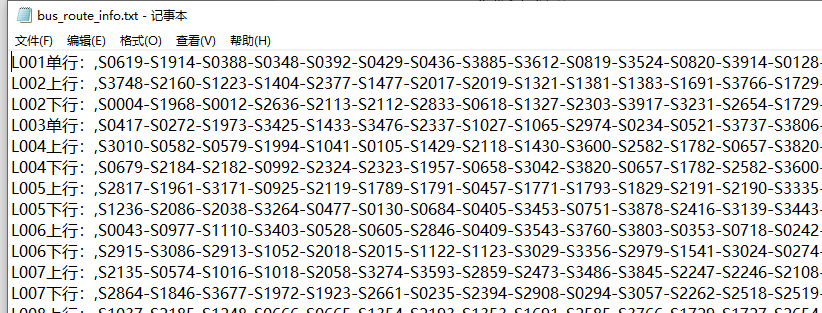题目要求：

请给出站点S1018-S2184的直达线路的查询结果
请给出站点S1771-S0886换乘一次线路的查询结果，并分析查询所得线路方案的优劣

思路
写两个函数，函数1是查询搜索经过某一站点的所有线路，函数2是查询搜索某两条公交线路的公共站点。
对于问题1，我们只需要通过函数1得到包含起点站start和终点站end的所有线路start_routes和end_routes，然后取出相同的线路（既包括起点站又包括终点站）然后判断起点站和终点站在线路中的位置索引是否满足start_idx<end_idx即可。比如下表中假设我们得到了包含S1018的线路有L005,L010,L020三条，包含S2184的有四条。因为需要直达，因此我们必须选择左右相同的线路，即选择L005线路，同时需要判断S1018站在L005中的位置索引是否小于S2184的位置索引，只有满足这个关系才可以从S1018直达S2184。

S1018
S2184

L005
L008

L010
L005

L020
L008

-
L010

对于问题2，同样的我们需要通过函数1得到包含起点站start和终点站end的所有线路start_routes和end_routes，然后取出不同的线路（因为要换乘，所以一条线路不能同时包含起点站和终点站）然后利用函数2得到任意两条不同线路的公共站点（注意这里公共站点可能有多个），然后同样的判断位置索引关心即可。只是这里需要注意的是，这里因为是不同的两条线路，所以判断的时候要两边公共站点在线路1中的索引大于起点站索引且在线路2中的索引小于终点站索引。示意图如下：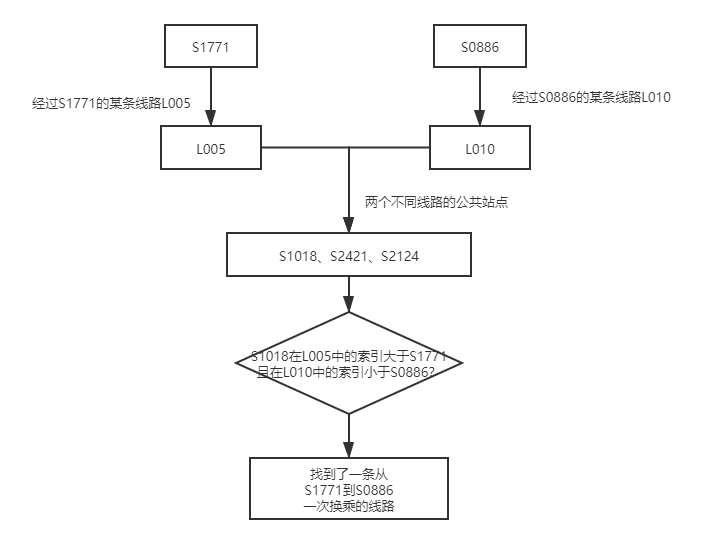代码
函数1：
//查询搜索经过某一站点的所有线路,idx是输入站点所在的线路索引和输入站点在所在线路中的位置
int get_all_routes(char *station, int idx)
{
int station_num, i, j, routes_num = 0;
char **ret;
for(i = 0; i < 36; i++)
{
ret = explode('-', bus_route[i], &station_num); //将当前线路切分后返回一个数组
for(j = 0; j < station_num; j++)
{
if( strcmp(ret[j], station) == 0 ) //判断当前线路是否经过输入站点
{
idx[routes_num] = i;
idx[routes_num] = j;
routes_num = routes_num + 1;
}
free(ret[j]);
}
free(ret);
}
return routes_num;
}

函数2：
//查询搜索给定两条线路的所有公共站点,common_idx是给定两条线路公共站点在各自线路中的位置
int get_common_station(int station_idx1, int station_idx2, int common_idx)
{
int i, j, ret1_num, ret2_num, common_num = 0;
char **ret1 = explode('-', bus_route[station_idx1], &ret1_num);
char **ret2 = explode('-', bus_route[station_idx2], &ret2_num);
for(i = 0; i < ret1_num; i++)
{
for(j = 0; j < ret2_num; j++)
{
if( strcmp(ret1[i], ret2[j]) == 0 )
{
common_idx[common_num] = i;
common_idx[common_num] = j;
common_num = common_num + 1;
}
}
}
free(ret1);
free(ret2);
return common_num;
}

主函数部分主要代码：
if( ch == 1 )
{   //定义所需要用到的数据类型
int i, start_num, end_num, routes_idx = 0;
int start_idx, end_idx;
char start_station, end_station;
char **routes = calloc(start_num + end_num, sizeof(char *));
//将矩阵初始化为全-1
init_matrix(36, 2, (int**)start_idx);
init_matrix(36, 2, (int**)end_idx);
//按照提示输入起点和终点
printf("请输入起点站：");
scanf("%s", start_station);
printf("请输入终点站：");
scanf("%s", end_station);
//使用函数get_all_routes计算包含起点和终点的所有线路的"索引"
start_num = get_all_routes(start_station, start_idx);
end_num = get_all_routes(end_station, end_idx);
//比较包含起点和终点线路中相同的线路，同时满足起点索引小于终点索引即可直达
for(i = 0; i < start_num; i++)
{
int j;
for(j = 0; j < end_num; j++)
{
if( start_idx[i] == end_idx[j] && start_idx[i] < end_idx[j] )
{
routes[routes_idx] = calloc(strlen(bus_route[start_idx[i]]) + strlen(bus_route[start_idx[i]]) + 1, sizeof(char));
memcpy(routes[routes_idx], bus_route[start_idx[i]], strlen(bus_route[start_idx[i]]) + 1);
strcat(routes[routes_idx], bus_route[start_idx[i]]);
routes_idx = routes_idx + 1;
}
}
}
//打印出直达线路，包括线路名称和具体线路路线
printf("从%s站到%s站的直达线路为：(查询结果为空则表示当前两站点没有直达线路)\n", start_station, end_station);
for(i = 0; i < routes_idx; i++)
{
puts(routes[i]);
printf("\n");
}

free(routes);

}

if( ch == 2 )
{
int common_idx, start_idx, end_idx;
int i, start_num, end_num, common_num, ret_num, routes_idx1 = 0, routes_idx2 = 0, common_station_idx = 0;
char start_station, end_station;
char **routes1 = calloc(100, sizeof(char *));
char **routes2 = calloc(100, sizeof(char *));
char **common_station = calloc(100, sizeof(char *));

init_matrix(100, 2, (int**)common_idx);
init_matrix(36, 2, (int**)start_idx);
init_matrix(36, 2, (int**)end_idx);

printf("请输入起点站：");
scanf("%s", start_station);
printf("请输入终点站：");
scanf("%s", end_station);

start_num = get_all_routes(start_station, start_idx);
end_num = get_all_routes(end_station, end_idx);

for(i = 0; i < start_num; i++)
{
int j;
for(j = 0; j < end_num; j++)
{
if( start_idx[i] != end_idx[j] )
{
common_num = get_common_station(start_idx[i], end_idx[j], common_idx);
int k;
for(k = 0; k < common_num; k++)
{
if( start_idx[i] < common_idx[k] && common_idx[k] < end_idx[j])
{
routes1[routes_idx1] = calloc(strlen(bus_route[start_idx[i]]) + strlen(bus_route[start_idx[i]]) + 1, sizeof(char));
memcpy(routes1[routes_idx1], bus_route[start_idx[i]], strlen(bus_route[start_idx[i]]) + 1);
strcat(routes1[routes_idx1], bus_route[start_idx[i]]);
routes_idx1 = routes_idx1 + 1;

common_station[common_station_idx] = explode('-', bus_route[start_idx[i]], &ret_num)[common_idx[k]];
common_station_idx = common_station_idx + 1;

routes2[routes_idx2] = calloc(strlen(bus_route[end_idx[j]]) + strlen(bus_route[end_idx[j]]) + 1, sizeof(char));
memcpy(routes2[routes_idx2], bus_route[end_idx[j]], strlen(bus_route[end_idx[j]]) + 1);
strcat(routes2[routes_idx2], bus_route[end_idx[j]]);
routes_idx2 = routes_idx2 + 1;
}
}
}
}
}

printf("从%s站到%s站的一站换乘线路为：(查询结果为空则表示当前两站点没有一站换乘线路)\n", start_station, end_station);
for(i = 0; i < routes_idx1; i++)
{
printf("第一程%s", routes1[i]);
printf("-------在%s站下车同站换乘--------", common_station[i]);
puts(routes2[i]);
printf("\n");
}

free(routes1);
free(routes2);
free(common_station);

}

运行结果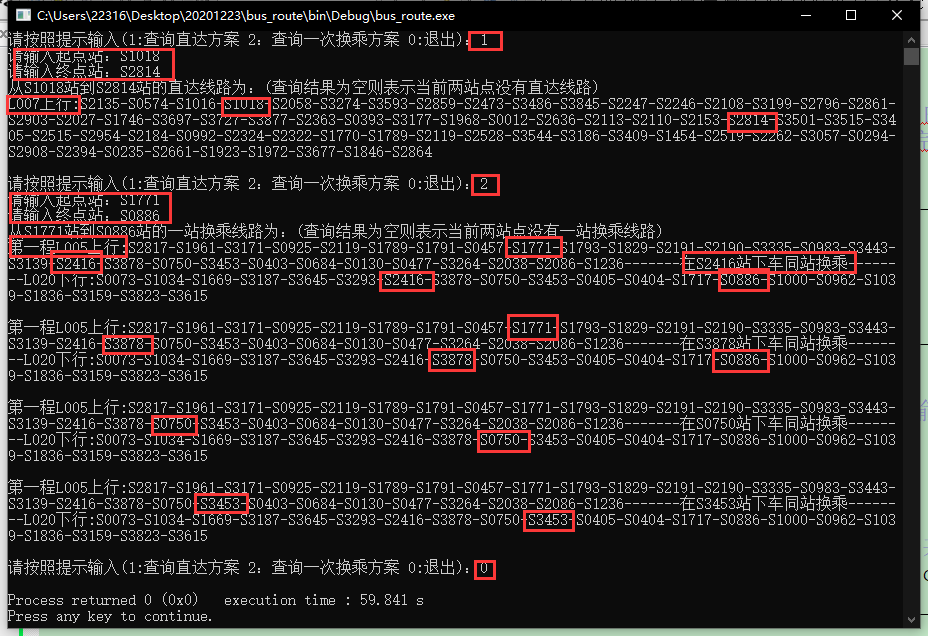其他
由于C不想python这种抽象度比较高的语言有很多现成的API可以用，因此许多功能需要自己实现一下，除了上边写到的比较核心的代码，文中还用到了：

一个是生成一个全为某个值的矩阵

//将矩阵初始化为值全为-1
void init_matrix(int row, int column, int **matrix)
{
int i, j;
for(i = 0; i < row; i++)
{
for(j = 0; j < column; j++)
{
*((int*)matrix + column*i + j) = -1;
}
}
}

如何调用前边的main代码里有

一个是制定字符进行切割，这里网上有很多，我这里直接copy了这位大哥的，有兴趣可以看原文，下边把代码也放上

//返回一个 char *arr[], size为返回数组的长度,该函数用于切割字符串
char **explode(char sep, const char *str, int *size)
{
int count = 0, i;
for(i = 0; i < strlen(str); i++)
{
if (str[i] == sep)
{
count ++;
}
}

char **ret = calloc(++count, sizeof(char *));

int lastindex = -1;
int j = 0;

for(i = 0; i < strlen(str); i++)
{
if (str[i] == sep)
{
ret[j] = calloc(i - lastindex, sizeof(char)); //分配子串长度+1的内存空间
memcpy(ret[j], str + lastindex + 1, i - lastindex - 1);
j++;
lastindex = i;
}
}
//处理最后一个子串
if (lastindex <= strlen(str) - 1)
{
ret[j] = calloc(strlen(str) - lastindex, sizeof(char));
memcpy(ret[j], str + lastindex + 1, strlen(str) - 1 - lastindex);
j++;
}

*size = j;

return ret;
}

同样如何调用主函数里都有

哦对了还有如何读取文件的函数…

//读入文件函数
void read_bustxt(char *file)
{
int i,split_num;
char mybus_route;
char **ret = calloc(300, sizeof(char *));
FILE * fp;
//读取文件
if((fp=fopen(file,"r"))==NULL)
{
printf("cannot open file\n");
exit(0);
}
for(i=0;i<100;i++)                        // 设定 文件公交线路信息.txt 有300行
{
fscanf(fp,"%s",mybus_route[i]);                         //读文件的每一行

if(strcmp(mybus_route[i],"END")==0)
break;                       //判断是否结束
}
fclose(fp);
for(i=0;i<36;i++)
{
ret = explode(',', mybus_route[i], &split_num);
bus_route[i] = ret;
bus_route[i] = ret;
}
free(ret);
}

结语
总之写的很粗糙，给以后还有需要的学弟们一点小小帮助吧。。。另外如果需要整个完整项目代码的话，点这里。
或者xingaoli020@foxmail.com


展开全文• 序设计说明书与实验报 告 C 语 言 程 序 设 计 实验报告 学院资源加工与生物工程 专业无机非金属材料工程 班级0501 姓名金光 学号0302050107 指导老师金胜明 制作日期 05 年 7 月 9 日 C 语言程序设计说明书 ...
• 图书管理系统C语言程序设计实验分析报告C语言程序设计实验报告系别专业班级姓名学号时间实验题目 图书信息管理系统一、实验目的(1)熟练掌握C语言的相关操作。(2)综合应用C语言的知识，独立完成一个完整的系统，提高...
• C 语 言 程 序 设 计 实验报告 学院资源加工与生物工程 专业无机非金属材料工程 班级 0501 姓名金光龙 学号 0302050107 指导老师金胜明 制作日期 05 年 7 月 9 日 C 语言程序设计说明书 目录 一 程序设计目的及任务 ...
• 4.掌握书写程序设计说明文档的能力 5.提高运用C语言解决实际问题的能力 二.程序要求 学生信息包括：学号，姓名，班级，地址，电话，E-mail等。设计一个学生通讯录管理系统，应具有以下功能： 1、学生通讯信息录入...数据库 vc++
• C语言实验七 数 实验报告C语言程序设计实验报告实验题目实验七 函数实验目的掌握函数定义的方法；掌握函数实参与形参的对应关系，以及值传递的方式。掌握函数的嵌套调用和递归调用的方法；掌握全局变量和局部变量、...
• 实验要求 C语言读盘程序设计块方式或文件方式(电子版) (1.写的代码要有注释说明 (2.要有运行结果截图 (3.以实验报告的形式提交 ?输入:磁头所在的位置以及对块的请求序列 ?输出:对块的调度访问序列 ?算法:你自己用...
• 该楼层疑似违规已被系统折叠隐藏此楼查看此楼加解密处理1、系统的基本功能编写一个对文件(由数字或字母组成)进行加密解密的程序。可以将所需要的内容(整个文件或者输入的一行字符)加密，也可以将存储的加密文件翻译...
• 华中科技大学计算机科学与技术学院 C 语言程序设计课程设计 基础部分实验报告 题目科技成果信息管理系统 专业计算机科学与技术 目录 一系统功能结构设计 1 二数据结构设计 1 三程序结构 4 四函数原型及功能 9 五实验...
• 实验要求 C 语言读盘程序设计块方式或文件方式 ( 电子版 ) (1. 写的代码要有注释说明 (2. 要有运行结果截图 (3. 以实验报告的形式提交 输入 :磁头所在的位置以及对块的请求序列 输出 :对块的调度访问序列 算法 :你...
• 实验要求 C 语言读盘程序设计块方式或文件方式 (电子版 ) (1. 写的代码要有注释说明 (2. 要有运行结果截图 (3. 以实验报告的形式提交 输入 :磁头所在的位置以及对块的请求序列 输出 :对块的调度访问序列 算法 :你...
• 高级程序语言设计实验C语言文件操作-16点阵字符显示.docx 西南交通大学 报告仅供参考，请独立完成作业
• 设计一图书信息管理系统，使之能提供以下功能： 2.系统以菜单方式工作 3.图书信息录入功能(图书信息用文件保存)－－输入 4.图书信息浏览功能－－输出 5.查询和排序功能：(至少一种查询方式)－－算法 6.按...图书管理系统
• 哈夫曼树实验报告 需求分析 从终端读入一串字符 利用建立好的哈夫曼树对其进行编码 储存到文件当中去 然后从文件读入 哈夫曼编码针对每个字母对其进行译码翻译为原来的信息 二概要设计 程序分为以下几个模块 1从终端...
• 该资源是我自己 在大一下 学期编写的c语言程序设计与算法课程设计》 综合性、设计性实验。该课设实验用的是c语言编写，编写的题目是《班级财务管理程序》。 设计一个班级财务管理系统，该程序具有以下功能： （1...
• C语言程序设计实验报告（学生成绩管理系统），使用C语言进行编写程序代码，环境为vc6.0。程序要求： 1) 具有菜单，可以在菜单上进行选择； 2) 能实现学生数据录入、修改、删除、查询； 3) 能实现数据排序； 4) 有...
• 实用标准文案 C 语言程序设计 实验报告 班级 CS1108 日期 2012/5/26 学生姓名 王一微 学号 U201114404 第 3 次上机 实验 1 编译预处理 ( 一) 实验目的 1掌握文件包含宏定义条件编译 assert 宏的使用 2 练习带参数的...
• 了解常用的C语言程序设计方法和组成部分。 2．学习编制连接命令文件，并用来控制代码的连接。 3．学会建立和改变map文件，以及利用它观察DSP内存使用情况的方法； 4．熟悉使用软件仿真方式调试程序。# c语言程序设计文件实验报告c语言 订阅